## Worksheet 06a: Strange Residuals

This page makes much more sense if you have read and understood Worksheet 06 in that here we build off some of the data from that worksheet. This time, however,we cannot use `gnrnd4()` to generate the data. In fact, for this worksheet we will start with all of the commands that we wil use:
```new_L1 <- c(9.6,15.5,25,9.6,-8.3,1.7,7.3,10.2,
-2.2,20.3,9.8,25.5,15.3,18.1,0.9,10.3,
3.8,13.3,8.9,7.7,2.8,-5.4,-4.7,9.5,23.1,
2.2,22.5,14.9,14.7,24.2)
new_L2 <- c(-9.9,-25.2,-47,-12.3,24.6,4.1,-7.3,-13.8,
12.1,-35.5,-12.8,-37.7,-24.7,-30.9,5.7,-11,
-0.2,-16.9,-10.7,-6.3,1.8,19.4,18.1,-9.8,
-33.8,4.5,-33.7,-23.9,-19.4,-35.9)
plot(new_L1,new_L2)
ws06a_lm <- lm(new_L2~new_L1)
ws06a_lm
summary( ws06a_lm)
abline( ws06a_lm, col="red", lwd=2)
cor(new_L1,new_L2)
new_resid <- residuals( ws06a_lm )
plot(new_L1,new_resid)
```

We will follow the usual procedure for doing our work on the USB drive. In particular, we have
1. inserted our USB drive,
2. created a directory called `worksheet06a` on that drive
3. have copied `model.R` from our root folder into our new folder,
4. have renamed that new copy of the file to the name `ws06a.R`, and
5. have double clicked on that file to open RStudio.
The result should be a window pretty much identical to the one shown in Figure 1.

Figure 1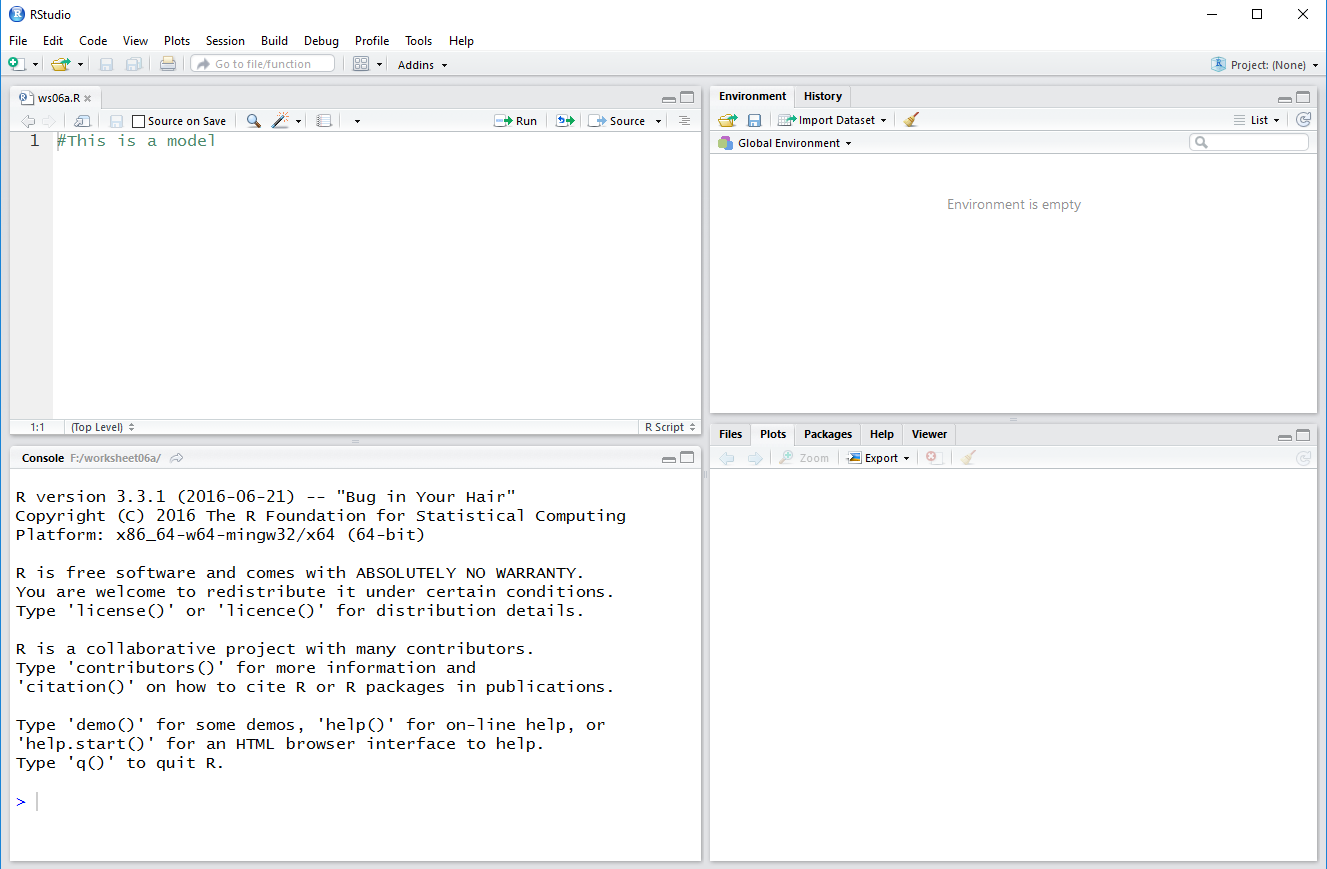Then, we highlight the commands given above on this we page as shown in Figure 1a.

Figure 1a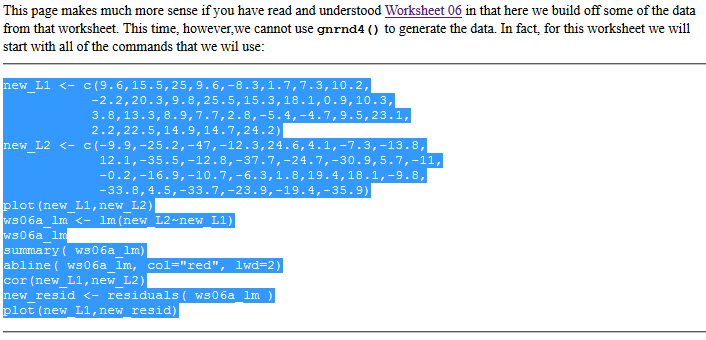We copy those commands (via the Ctrl-C key sequence on a Windows machine) and paste them (via a Ctrl-V key sequence) into our `ws06a.R` worksheet in our RStudio session Editor pane. This should produce the image shown in Figure 1b.

Figure 1b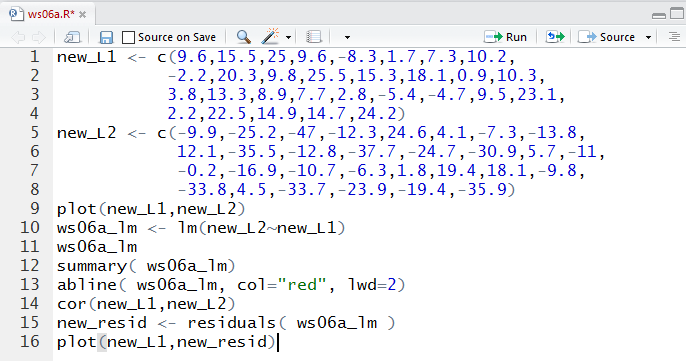Now that we have all of the commands we can highlight the ones we want to execute and then run those commands. Figure 2 shows the two commands to put values into the variables `new_L1` and `new_L2` plus the command to create a scatter plot of the values in those two variables.

Just to help us understand the relationship of these values to the ones we reated in Worksheet 06, you might observe that the `new_L1` values are identical to those in `L1` in the old example. The values in `new_L2` have changed, slightly, but they are not far off from the values that we had.

Figure 2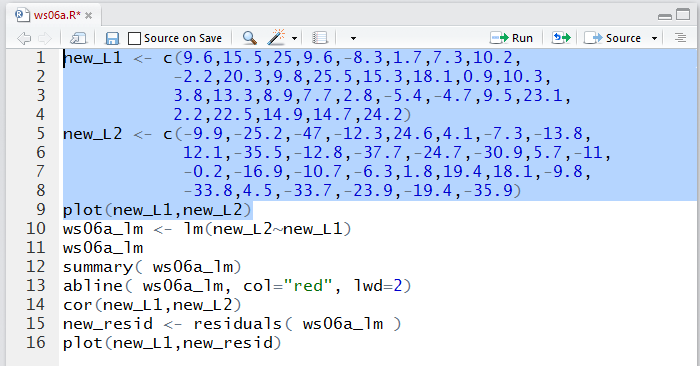Running the lines highlighted in Figure 2produces the plot in Figure 3.

Figure 3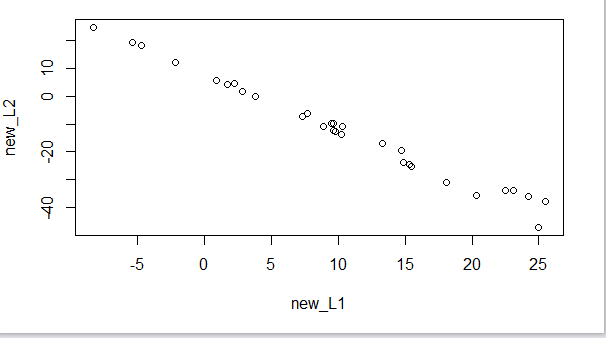The similarity in the values we have in Figure 3 to those that we had for Worksheet 06 is brought home when we go back and look at the scatter plot from that example shown again in Old Figure 6.

Old Figure 6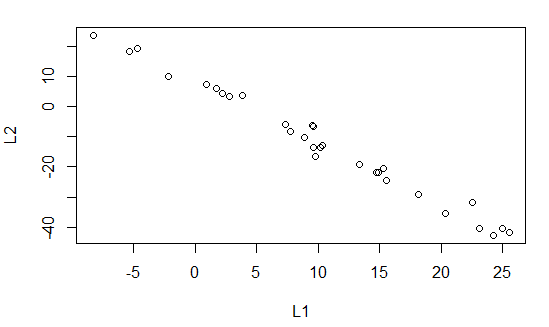Moving on we create a linear model for the relation between `new_L1` and `new_L2`. Those are the commands in lines 10 and 11 of our list of commands.

Figure 4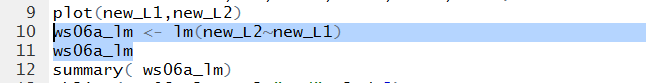The output, shown in Figure 5, gives rise to the regression equation
`y =7.753 + (-1.963)x`
This is indeed slightly different from the line we got in the previous worksheet, but not much different.

Figure 5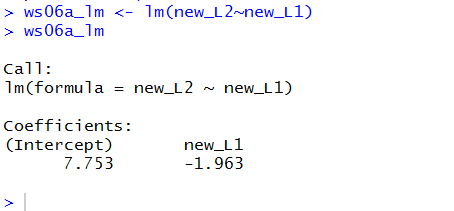The `summary(ws06a_lm)` command gives us even more information about our linear model.

Figure 6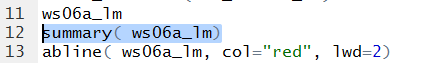The result of running the command is given in Figure 7.

Figure 7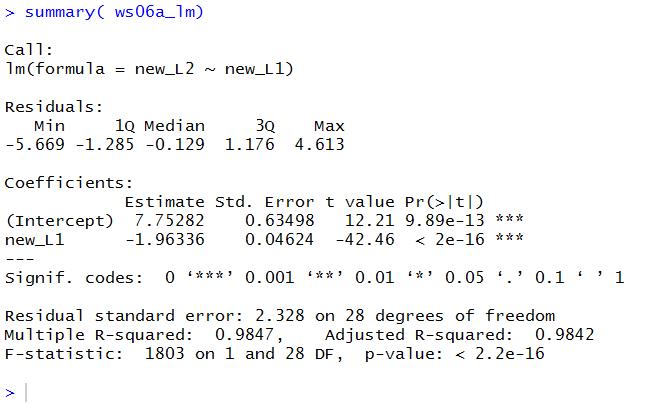We can compare the results shown in Figure 7 with those that we saw in the earlier example, shown here in Old Figure 15.

Old Figure 15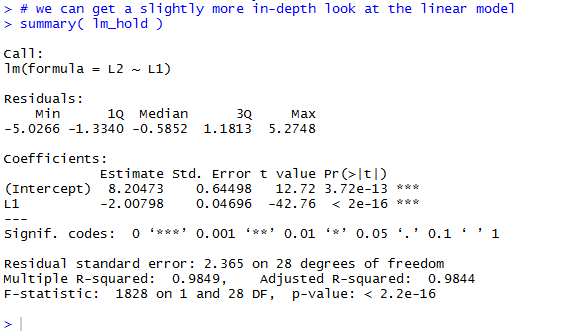Again, we can detect differences, but they seem minor.

Figure 8 shows the command we need to add the regression line to the scatter plot.

Figure 8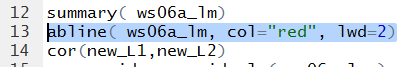Figure 9 shows that new graph.

Figure 9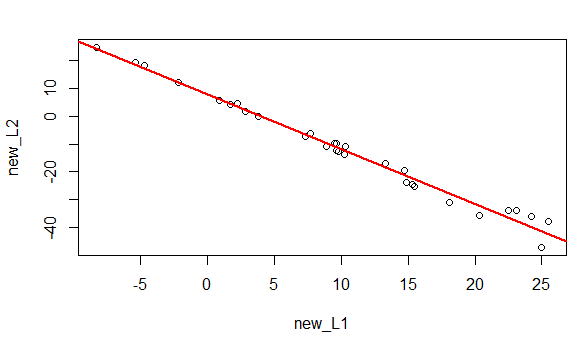Of course we want to find the correlation coefficient.

Figure 10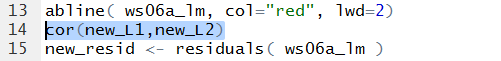/center> The value of the correlation coefficient is given in Figure 11. Again, this is not much different from the value in the earlier worksheet.

Figure 11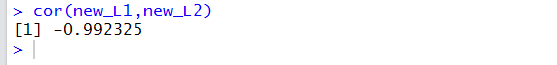But now we get to the whole point of this worksheet, wewill look at the residual values. Figure 12 holds the commands to retrieve those values from the linear model and then to plot them.

Figure 12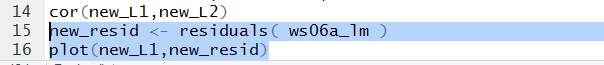As you should expect at this point, there is not much to see in the Console pane shown in Figure 13.

Figure 13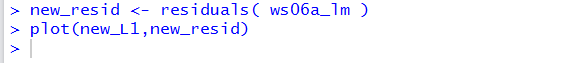However, the scatter plot shown in Figure 14 is the real change from the earlier example. Here the residual values are not all over the place. They form a definite, if strange, pattern. Seeing this would be enough to make us cautious about applying the linear regression equation. In particular, in this case, what we see is that as the x-values increase (as we move toward the right side of the graph) the residual values move further and further away from 0. In other words, for low values of x it appears that the regression equation is outstanding. For higher values it is not as good. We would be exceptionally cautious in extrapolating with values in excess of 25.5, our highest x-value.

Figure 14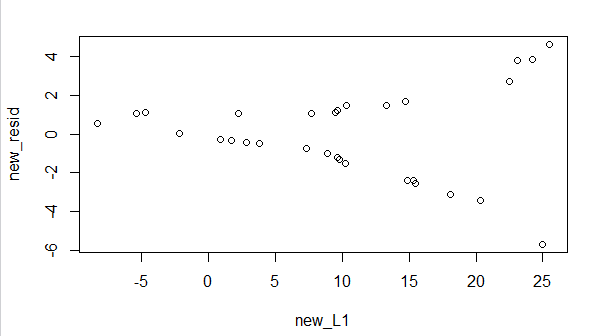Not shown here are the usual steps of saving our file and quiting our RStudio session.